# MCAT Biology : Reactions with Hydrocarbons

## Example Questions

### Example Question #11 : Reactions

The most stable radical shown below is __________. The least stable radical shown below is __________.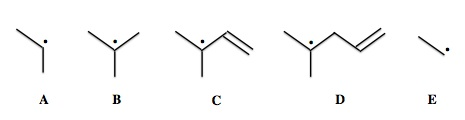Explanation:

Radical stability increases as carbon substitution increases. In addition, radicals in conjugation with double bonds via resonance are more stable than the corresponding non-conjugated radical.

In this case radicals B, C, and D are all tertiary radicals, but only radical C has additional stabilization from resonance. Radical C is therefore the most stable. Radical E is the least substituted of the five radicals, and is the least stable.

### Example Question #1 : Carbocations

What intermediate is involved in the conversion of compound B to compound C?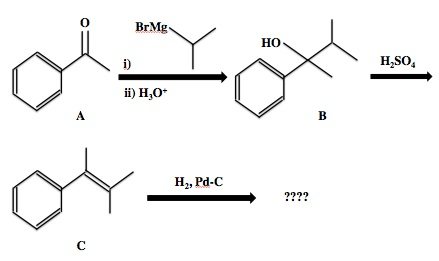Secondary carbocation

Tertiary carbanion

Tertiary carbocation

Tertiary carbocation

Explanation:

The strong sulfuric acid protonates the hydroxyl group of compound B, resulting in the loss of water as a leaving group and the generation of a carbocation intermediate. Since this carbocation carbon is attached to three other carbons, this is a tertiary carbocation. It is bound to the phenyl substituent, a methyl group, and the branched carbon chain.

### Example Question #11 : Reaction Types

The combustion of pentane with oxygen gas is an exothermic reaction that produces carbon dioxide and water as products. Is this a spontaneous reaction?

Yes, at all temperatures

Yes, only at low temperatures

No, never

Yes, only at high temperatures

Yes, at all temperatures

Explanation:

A balanced equation of the combustion of pentane indicates that one mole of pentane reacts with eight moles of oxygen gas to produce five moles of carbon dioxide and six moles of water.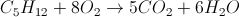Because there are nine moles of reactant and eleven moles of product, entropy increases in this reaction. Exothermic reactions that increase entropy are favorable at all temperatures, as seen in the Gibb's free energy equation.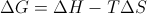In our scenario, H is negative and S is positive.

### Example Question #31 : Organic Chemistry, Biochemistry, And Metabolism

Which of the following statements concerning the combustion of cycloalkanes is false?

As the number of carbons in the ring increases, the molar heat of combustion increases

Oxygen is necessary in order for a combustion reaction to take place

The heat of combustion per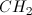in cyclohexane is greater than the heat of combustion perin cyclobutane

The molar heat of combustion for cyclooctane is nearly double the molar heat of combustion for cyclobutane

The heat of combustion perin cyclohexane is greater than the heat of combustion perin cyclobutane

Explanation:

The heat of combustion for cycloalkanes can be quantified based on two factors: the number of carbons in the ring, and the amount of strain in the ring. Cyclohexane does in fact have a larger molar heat of combustion than cyclobutane. This is because there are more carbons in the ring; however, ring stability will determine the heat of combustion per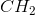group in the ring. Even though cyclohexane has more carbons than cyclobutane, the heat of combustion pergroup in cyclobutane will be greater compared to cyclohexane due to cyclobutane's ring strain.

### Example Question #4 : Reactions With Hydrocarbons

Which of the compounds shown above will have a greater heat of reduction (hydrogenation)?

Benzene

The compounds will have the same heat of reduction

1,3,5-hexatriene

1,3,5-hexatriene

Explanation:

The answer is 1,3,5-hexatriene. Chemicals that are more stable will give off less heat when they are reduced or hydrogenated. Although these two compounds contain the same number of pi bonds to be reduced, benzene is aromatic, and therefore is much more stable than the conjugated non-cyclic hexatriene. We would find that the heat of hydrogenation for hexatriene would be noticeably greater than that of benzene.

### Example Question #1 : Reactions With Hydrocarbons

Alkenes A through D contain only carbon and hydrogen. They have the following heats of combustion at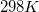.

Alkene A: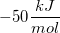Alkene B: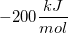Alkene C: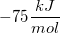Alkene D: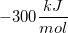Rank these four alkenes in terms of their stability, with the least stable compound on the left, and the most stable compound on the right.

A < D < B < C

A < C < B < D

D < C < B < A

D < B < C < A

A < B < C < D

D < B < C < A

Explanation:

A combustion reaction of any hydrocarbon yields the same products: carbon dioxide and water. The heat of combustion for the reaction shows how much energy is released as the hydrocarbon is converted to those products.

Since the products are the same for each alkene, any difference in heat of combustion will arise from differences in energy of the starting alkenes. Since the heat of combustion is negative, that means the reactants are higher in energy than the products, creating an exothermic reaction. Furthermore, the more negative the heat of combustion, the higher in energy the reactants are, and the less stable they are as well. Since compound D has the largest heat of combustion, it is highest in energy and therefore the least stable. By similar reasoning, B is the next highest in energy, followed by C, and then finally A, the most stable compound.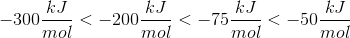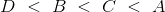### Example Question #41 : Organic Chemistry

Which of the following steps of free radical chlorination does not produce a free radical as a product?

Halogenation

Termination

Initiation

Propagation

Termination

Explanation:

The three steps of a free radical chlorination reaction are, in order, initiation, propagation, and termination.

Free radicals are produced in the initiation and propagation steps. The termination steps combine any two free radicals formed in the reaction to produce a compound that has no unpaired electrons (free radicals).

### Example Question #41 : Organic Chemistry, Biochemistry, And Metabolism

Consider the following radical bromination reaction of propane.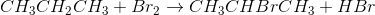Which of the following substances would NOT be a suitable radical initiator for this reaction?

Diisopropyl ether

Benzoyl peroxide

UV light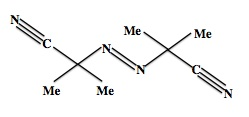Azobisisobutyronitrile (or AIBN)

tert-butyl peroxide